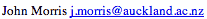Data Structures and Algorithms 3.4 Recursion

Many examples of the use of recursion may be found: the technique is useful both for the definition of mathematical functions and for the definition of data structures. Naturally, if a data structure may be defined recursively, it may be processed by a recursive function!
 recur From the Latin, re- = back + currere = to run To happen again, esp at repeated intervals.

### 3.4.1 Recursive functions

Many mathematical functions can be defined recursively:
• factorial
• Fibonacci
• Euclid's GCD (greatest common denominator)
• Fourier Transform
Many problems can be solved recursively, eg games of all types from simple ones like the Towers of Hanoi problem to complex ones like chess. In games, the recursive solutions are particularly convenient because, having solved the problem by a series of recursive calls, you want to find out how you got to the solution. By keeping track of the move chosen at any point, the program call stack does this housekeeping for you! This is explained in more detail later.

### 3.4.2 Example: Factorial

One of the simplest examples of a recursive definition is that for the factorial function:
```factorial( n ) = if ( n = 0 ) then 1
else n * factorial( n-1 )
```
A natural way to calculate factorials is to write a recursive function which matches this definition:

```function fact( int n )
{
if ( n == 0 ) return 1;
else return n*fact(n-1);
}```

Note how this function calls itself to evaluate the next term. Eventually it will reach the termination condition and exit. However, before it reaches the termination condition, it will have pushed n stack frames onto the program's run-time stack.

The termination condition is obviously extremely important when dealing with recursive functions. If it is omitted, then the function will continue to call itself until the program runs out of stack space - usually with moderately unpleasant results!

Failure to include a correct termination condition in a recursive function is a recipe for disaster!
Another commonly used (and abused!) example of a recursive function is the calculation of Fibonacci numbers. Following the definition:

```fib( n ) = if ( n = 0 ) then 1
if ( n = 1 ) then 1
else fib( n-1 ) + fib( n-2 )
```
one can write:

```function fib( int n )
{
if ( (n == 0) || (n == 1) ) return 1;
else return fib(n-1) + fib(n-2);
}```
Short and elegant, it uses recursion to provide a neat solution - that is actually a disaster! We shall re-visit this and show why it is such a disaster later.

Data structures also may be recursively defined. One of the most important class of structure - trees - allows recursive definitions which lead to simple (and efficient) recursive functions for manipulating them.

But in order to see why trees are valuable structures, let's first examine the problem of searching.

### Key terms

Termination condition
Condition which terminates a series of recursive calls - and prevents the program from running out of space for stack frames!

©, 1998